# Chapter 2 Title & Axis Labels

## 2.1 Introduction

In this chapter, we will learn how to add:

• Title
• Subtitle
• Axis Labels

to a plot and to modify:

• the range of an axis

In the previous chapter, we created plots which did not have any title or labels. Such plots are of no use to any one as they do not indicate what the X and Y axis represent or the primary information being communicated by the plot. The `title` and `labels` play an important part in making the plot holistic. There are two ways to add them to a plot:

• use the relevant arguments within the `plot()` function
• use the `title()` function

We will explore both the above methods one by one, and you can choose the method most convenient to you. Let us begin with the `plot()` function:

## 2.2 Syntax

Feature Argument Value Example
Title main string “Scatter Plot”
Subtitle sub string “Displacement vs Miles Per Gallon”
X Axis Label xlab string “Displacement”
Y Axis Label ylab string “Miles Per Gallon”

## 2.3 Title

You can add a title to the plot using the `main` argument in the `plot()` function. Ensure that the title is enclosed in single/double quotes as it is a string. Let us create a scatter plot of `disp` and `mpg` from `mtcars` data set, and add a title to it.

``````plot(mtcars\$disp, mtcars\$mpg,
main = 'Displacement vs Miles Per Gallon')``````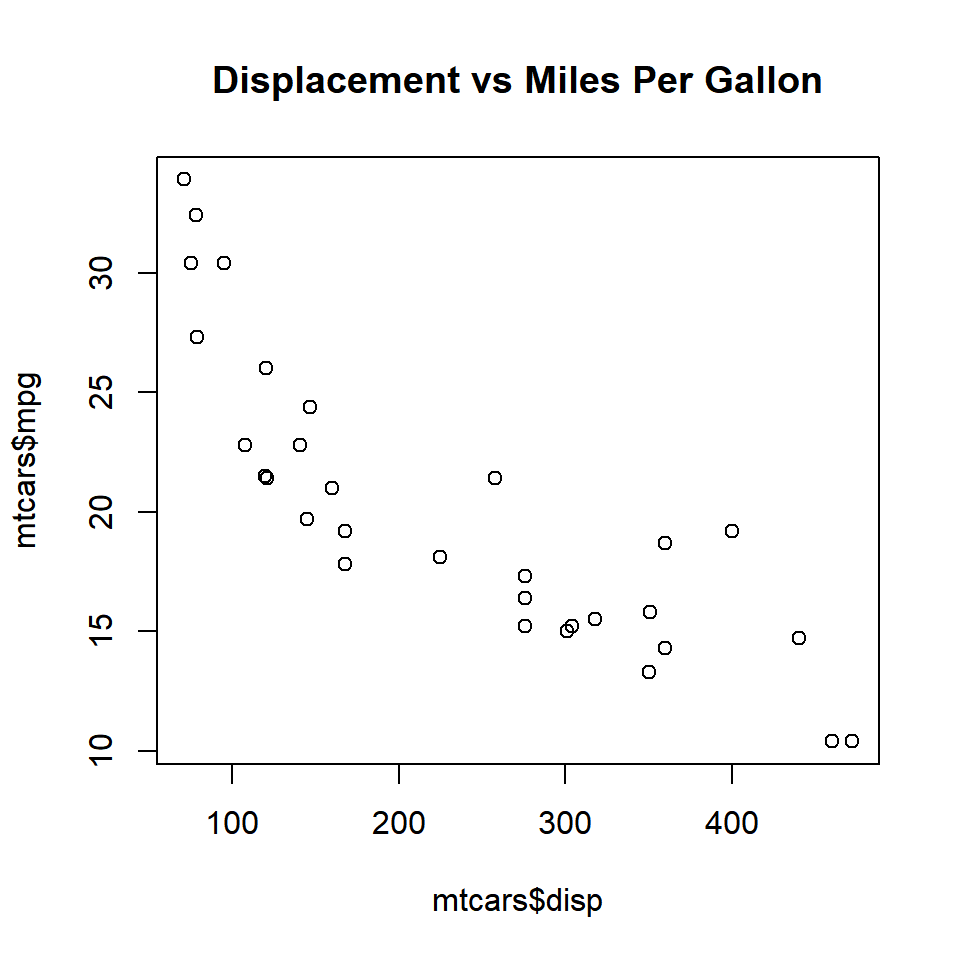## 2.4 Subtitle

You can add a subtitle to the plot using the `sub` argument in the `plot()` function. The subtitle will appear below the X axis label. Ensure that the subtitle is enclosed in single/double quotes as it is a string. Let us add a subtitle to the plot we created in the previous example:

``````plot(mtcars\$disp, mtcars\$mpg,
main = 'Displacement vs Miles Per Gallon',
sub = 'Mileage is inversely related to Displacement')``````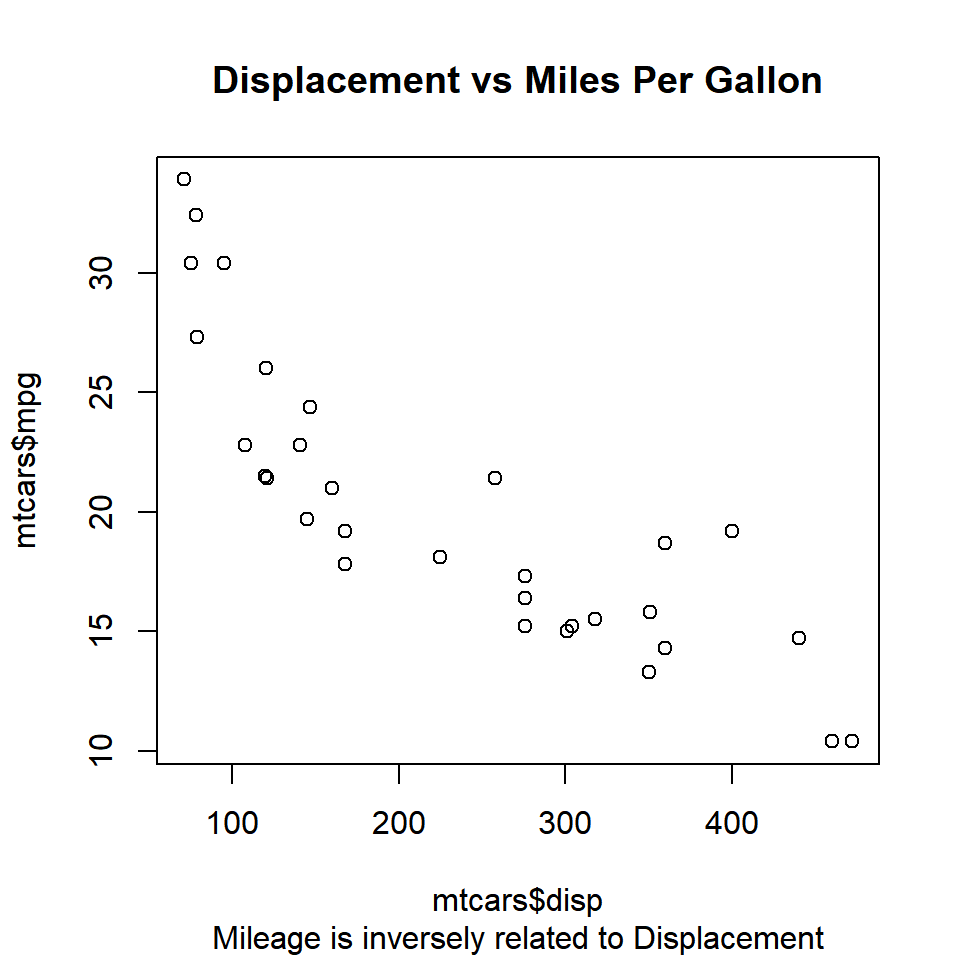## 2.5 Axis Labels

In the plots created in the previous examples, the axis labels appear as `mtcars\$mpg` and `mtcars\$disp`. It is not the best way to name the axis and it will make more sense to use names that describe the data. Let us modify the axis labels using the `xlab` and `ylab` arguments in the `plot()` function:

``````plot(mtcars\$disp, mtcars\$mpg,
main = 'Displacement vs Miles Per Gallon',
sub = 'Mileage is inversely related to Displacement',
xlab = 'Displacement', ylab = 'Miles Per Gallon')``````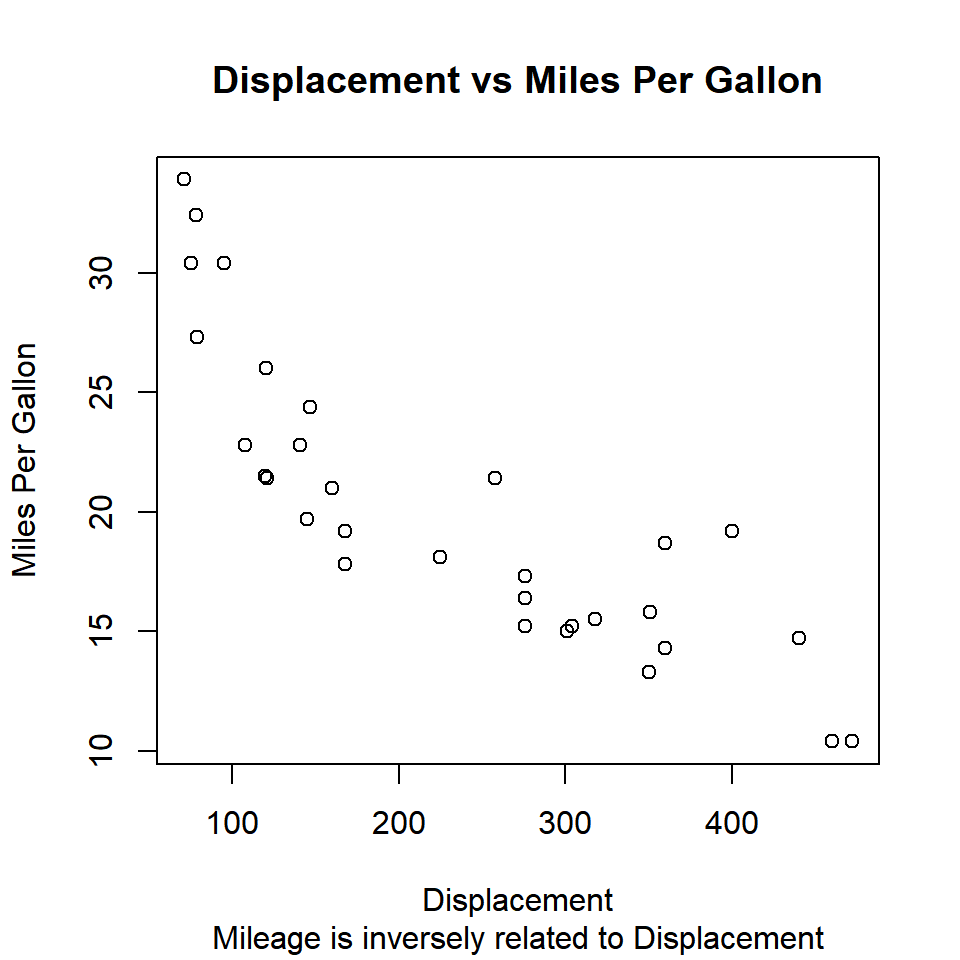## 2.6 title()

We can add title, subtitle and axis labels using the `title()` function as well. Let us recreate the plots from the previous examples but this time we will use the `title()` instead of the `plot()` function. We will continue to use the `plot()` function to create the plot.

``````# create scatter plot
plot(mtcars\$disp, mtcars\$mpg)

# add title, subtitle and axis labels
title(main = 'Displacement vs Miles Per Gallon',
sub = 'Mileage is inversely related to Displacement',
xlab = 'Displacement', ylab = 'Miles Per Gallon')``````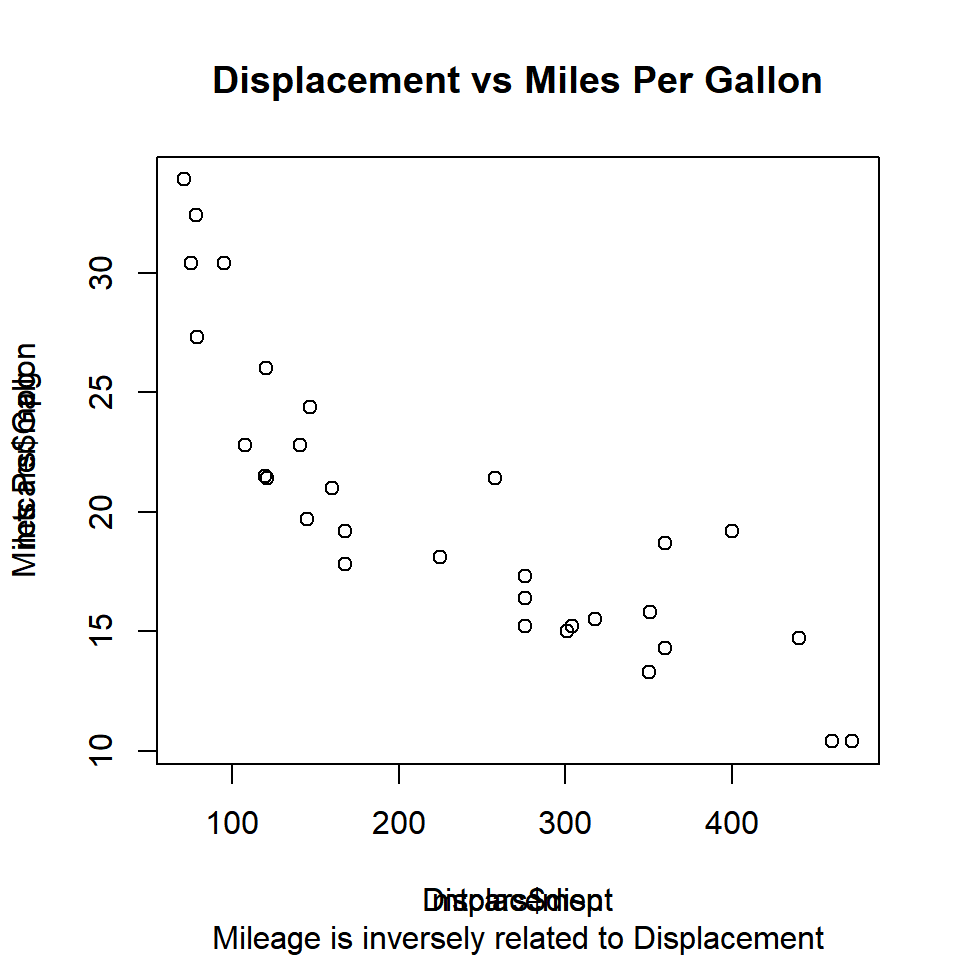Do you notice that the axis labels are overwritten? This happens because the `plot()` function adds the default labels and we add a new set of labels without modifying the existing ones. The solution is to instruct the `plot()` function not to add any labels to the X and Y axis. This can be achieved using the `ann` (annotate) argument in the `plot()` function and set it to `FALSE`. Let us try it:

``````# create scatter plot
plot(mtcars\$disp, mtcars\$mpg, ann = FALSE)

# add title, subtitle and axis labels
title(main = 'Displacement vs Miles Per Gallon',
sub = 'Mileage is inversely related to Displacement',
xlab = 'Displacement', ylab = 'Miles Per Gallon')``````The axis labels are legible and not overwritten. You can use either the `plot()` function or the `title()` function to add title, subtitle and axis labels but ensure that in case you use the `title()` function, set `ann` argument to `FALSE` in the `plot()` function.

## 2.7 Axis Range

In certain cases, you would want to modify the range of the axis of the plots. By default, the `plot()` function will take into account the `min` and `max` values of the variable(s) and set the range for the axis. We can modify the range by using the `xlim` and `ylim` arguments in the `plot()` function. Both the `xlim` and `ylim` arguments take 2 values as inputes. The first value is the minimum value for the axis and the second value is the maximum value for the axis. The `plot()` function will return an error if we do not specify two values for both `xlim` and `ylim` arguments. Let us recreate the plot from the previous examples but change the range of both the X and Y axis:

``````plot(mtcars\$disp, mtcars\$mpg,
xlim = c(0, 600), ylim = c(0, 50))``````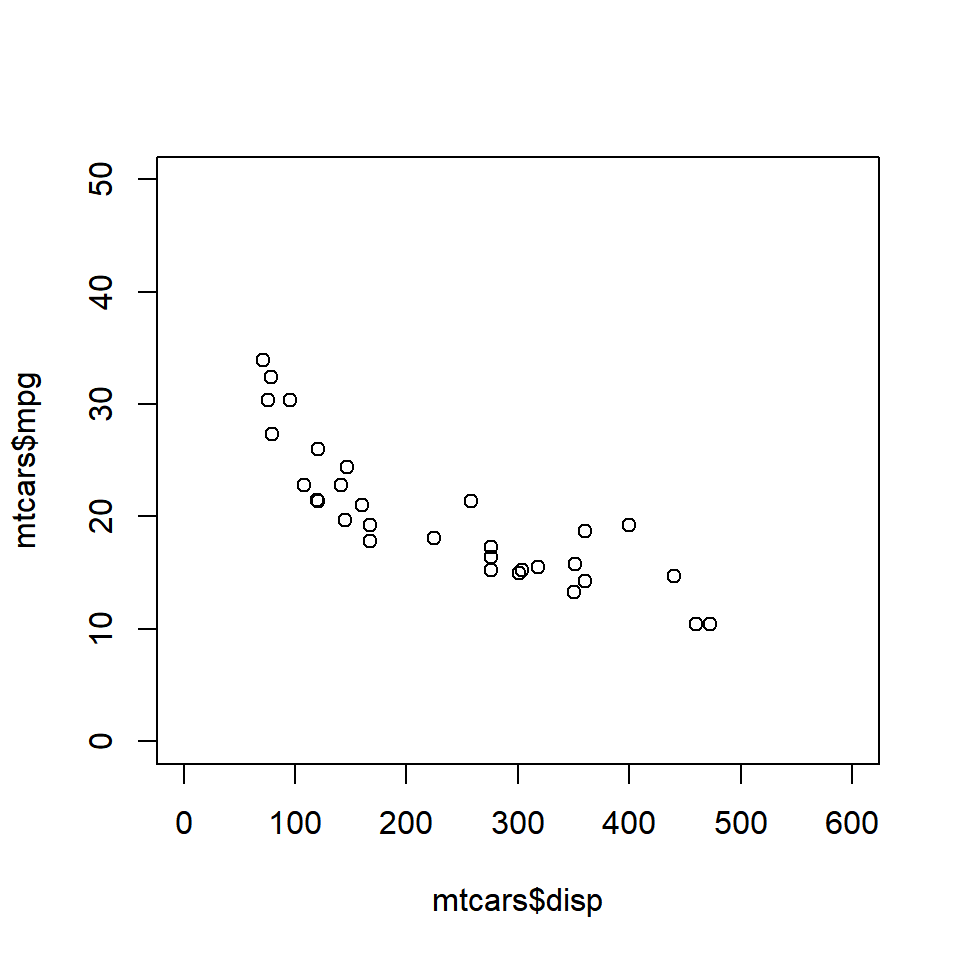Keep in mind that the axis ranges cannot be modified using the `title()` function.